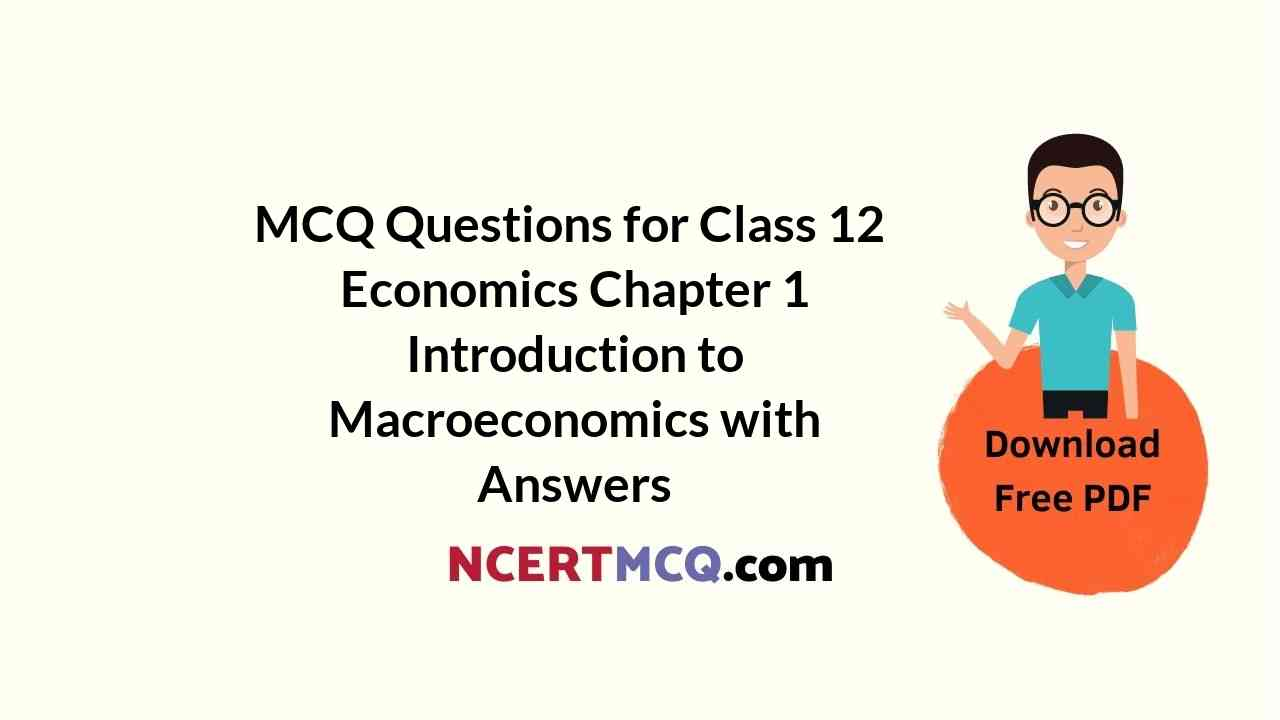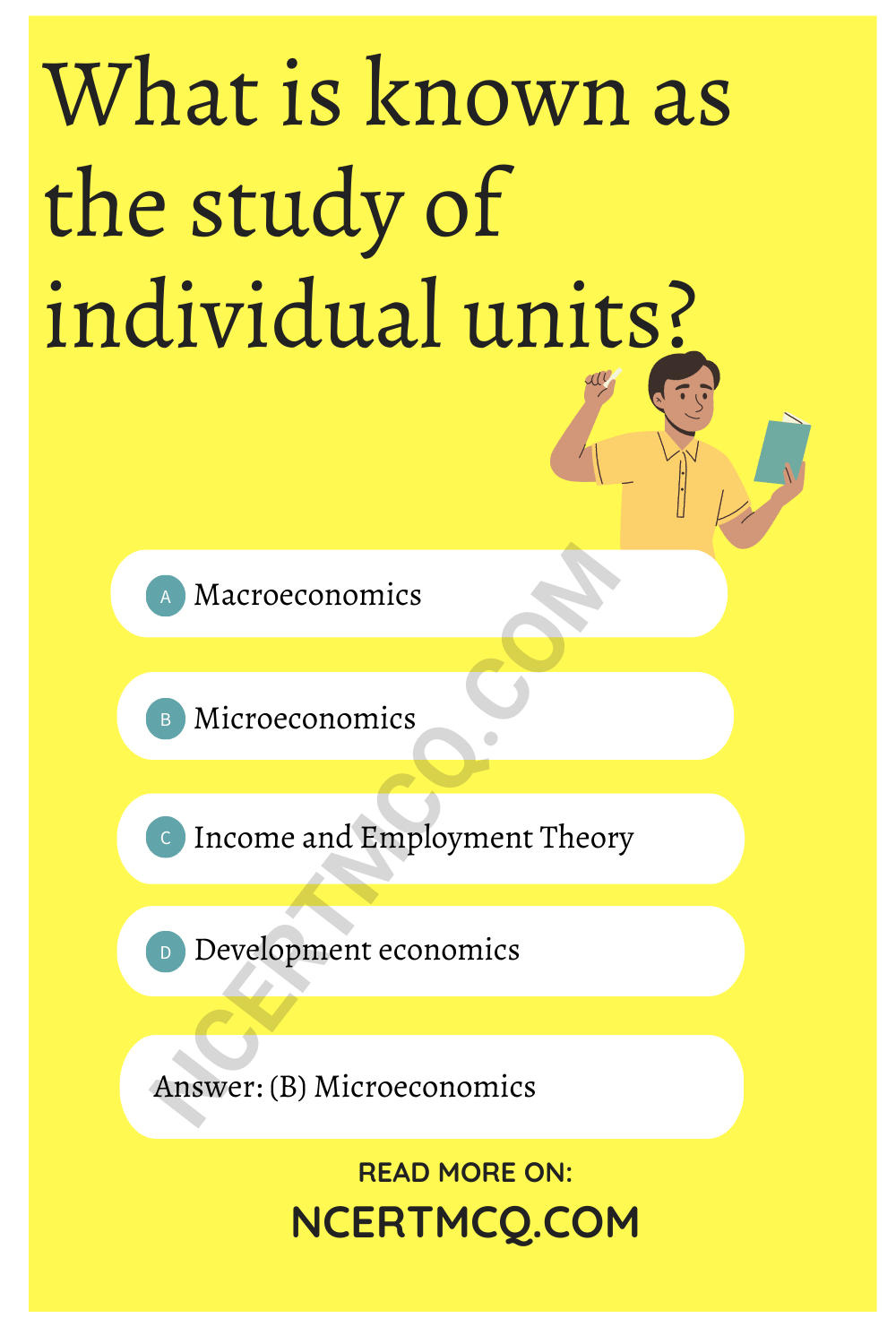Check the below NCERT MCQ Questions for Class 12 Economics Chapter 1 Introduction to Macroeconomics with Answers Pdf free download. MCQ Questions for Class 12 Economics with Answers were prepared based on the latest exam pattern. We have provided Introduction to Macroeconomics Class 12 Economics MCQs Questions with Answers to help students understand the concept very well.

## Introduction to Macroeconomics Class 12 MCQs Questions with Answers

I. Choose the correct alternative.

MCQ Questions For Class 12 Economics Chapter 1 Question 1.
What is known as the study of individual units?
(A) Macroeconomics
(B) Microeconomics
(C) Income and Employment Theory
(D) Development economicsMacroeconomics Class 12 Chapter 1 MCQ Question 2.
What is the main cause of all economic problems?
(A) Abundance
(B) Convenience
(C) Scarcity
(D) None of these

MCQ Class 12 Economics Chapter 1 Question 3.
Study of aggregates is known as _________
(A) Macroeconomics
(B) Microeconomics
(C) Price theory
(D) Factor price determination

Class 12 Economics Chapter 1 MCQ Question 4.
What is the name of the book written by J.M. Keynes?
(A) Wealth of Nation
(B) Political economy
(C) The General Theory of Employment, Interest and Money
(D) None of these

Answer: (C) The General Theory of Employment, Interest and Money

Macroeconomics MCQs With Answers Question 5.
When was the famous book of Prof. Keynes published?
(A) 1930
(B) 1931
(C) 1936
(D) 1940

Economics Class 12 Chapter 1 MCQ Question 6.
When did the great depression occur?
(A) 1929-30
(B) 1934-35
(C) 1938-39
(D) 1941-42

Class 12 Economics Ch 1 MCQ Question 7.
Who is known as the father of modern macroeconomics?
(B) J.M.Keynes
(C) Samuelson
(D) Hicks

Economics Chapter 1 Class 12 MCQ Question 8.
Mr. Skund Kumar wants to study the national income. Which branch of economics will he have to study?
(A) Microeconomics
(B) Price theory
(C) Factor price determination
(D) MacroeconomicsII. Fill in the blanks with the correct answer.

MCQ On Micro And Macro Economics Question 1.
The mother of all economic problems is _________

Class 12 Macroeconomics Chapter 1 MCQ Question 2.
Macroeconomics is defined as that branch of economics, which studies economic activities of an economy as a _______

MCQ Of Economics Class 12 Chapter 1Question 3.
_______ was the founding father of modern economics.

Introduction To Macroeconomics Class 12 MCQ Question 4.
Keynes book on Income and Employment came into light in the year _________

MCQ Of Chapter 1 Macroeconomics Class 12 Question 5.
Aggregate Demand is the sum total of consumption and _________

Question 6.
The period of the great depression was from __________

III. State whether the following statements are true or false.

Question 1.
Capital, land, labour, and entrepreneur are the factors of production.

Question 2.
The expenses which raise productive capacity are known as investment expenditure.

Question 3.
The domestic country may sell goods to the rest of the world. It is called imports.

Question 4.
The domestic country may buy goods from the rest of the world. It is called exports.

Question 5.
The capitalist economy works for social welfare.

Question 6.
Microeconomics deals with the aggregate economic variable of an economy.

Question 7.
‘Save more’ is always a virtue.

IV. Match the following.

Question 1.

 Column-I Column-II 1. Worldwide Depression (A) 1936 2. Publication year of Keynes’s book (B) 1929-30 3. Problem of unemployment (C) Microeconomics study 4. Study of the sugar industry (D) J.M.Keynes 5. Father of Macroeconomics (E) Ragnar Frisch 6. Who divided economics into two parts? (F) Macroeconomics study